#### Simplifying knots

A knot is a closed curve that does not intersect itself, is embedded in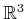, and cannot be untangled to produce a simple loop (such as a circular path). If the knot is allowed to intersect itself, then any knot can be untangled; therefore, a careful definition of what it means to untangle a knot is needed. For a closed curve,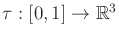, embedded in(it cannot intersect itself), let the set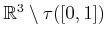of points not reached by the curve be called the ambient space of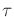. In knot theory, an ambient isotopy between two closed curves,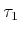and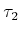, embedded inis a homeomorphism between their ambient spaces. Intuitively, this means thatcan be warped intowithout allowing any self-intersections. Therefore, determining whether two loops are equivalent seems closely related to motion planning. Such equivalence gives rise to groups that characterize the space of knots and are closely related to the fundamental group described in Section 4.1.3. For more information on knot theory, see [8,451,511].

A motion planning approach was developed in  to determine whether a closed curve is equivalent to the unknot, which is completely untangled. This can be expressed as a curve that maps onto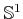, embedded in. The algorithm takes as input a knot expressed as a circular chain of line segments embedded in. In this case, the unknot can be expressed as a triangle in. One of the most challenging examples solved by the planner is shown in Figure 7.31. The planner is sampling-based and shares many similarities with the RDT algorithm of Section 5.5 and the Ariadne's clew and expansive space planners described in Section 5.4.4. Since the task is not to produce a collision-free path, there are several unique aspects in comparison to motion planning. An energy function is defined over the collection of segments to try to guide the search toward simpler configurations. There are two kinds of local operations that are made by the planner: 1) Try to move a vertex toward a selected subgoal in the ambient space. This is obtained by using random sampling to grow a search tree. 2) Try to delete a vertex, and connect the neighboring vertices by a straight line. If no collision occurs along the intermediate configurations, then the knot has been simplified. The algorithm terminates when it is unable to further simplify the knot.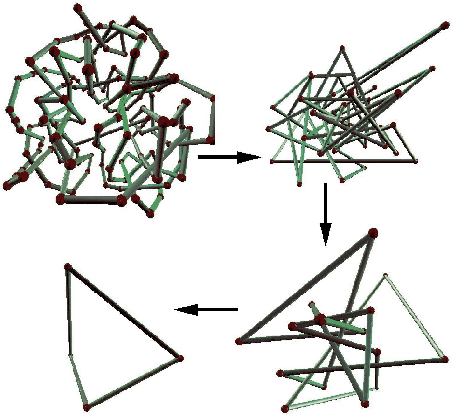Steven M LaValle 2020-08-14